# Basic concepts of C Presented by Prof Satyajit

• Slides: 14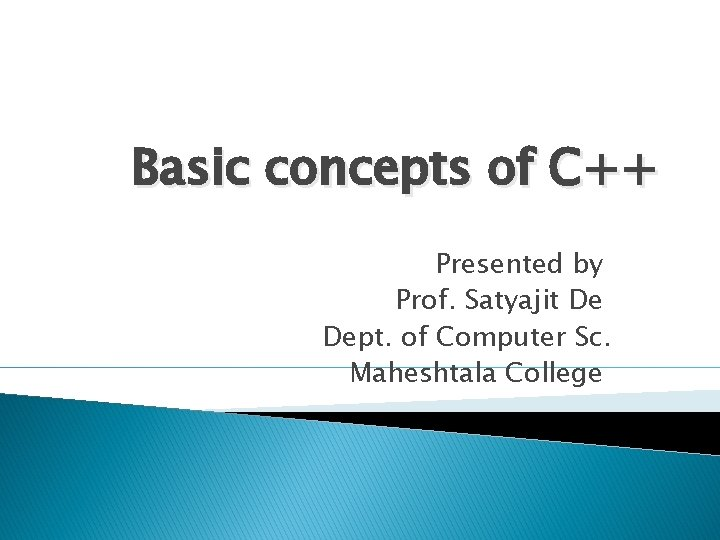Basic concepts of C++ Presented by Prof. Satyajit De Dept. of Computer Sc. Maheshtala College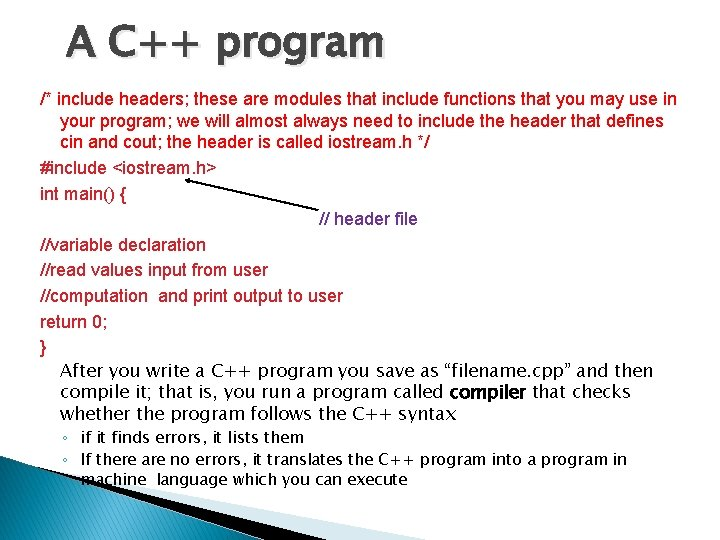A C++ program /* include headers; these are modules that include functions that you may use in your program; we will almost always need to include the header that defines cin and cout; the header is called iostream. h */ #include <iostream. h> int main() { // header file //variable declaration //read values input from user //computation and print output to user return 0; } After you write a C++ program you save as “filename. cpp” and then compile it; that is, you run a program called compiler that checks whether the program follows the C++ syntax ◦ if it finds errors, it lists them ◦ If there are no errors, it translates the C++ program into a program in machine language which you can executeNotes � � what follows after // on the same line is considered comment indentation is for the convenience of the reader; compiler ignores all spaces and new line ; the delimiter for the compiler is the semicolon all statements ended by semicolon Lower vs. upper case matters!! ◦ Void is different than void ◦ Main is different that mainSimple c++ program Here is the Hello world program in C++. #include <iostream. h> int main() { cout << “Hello world!”; return 0; }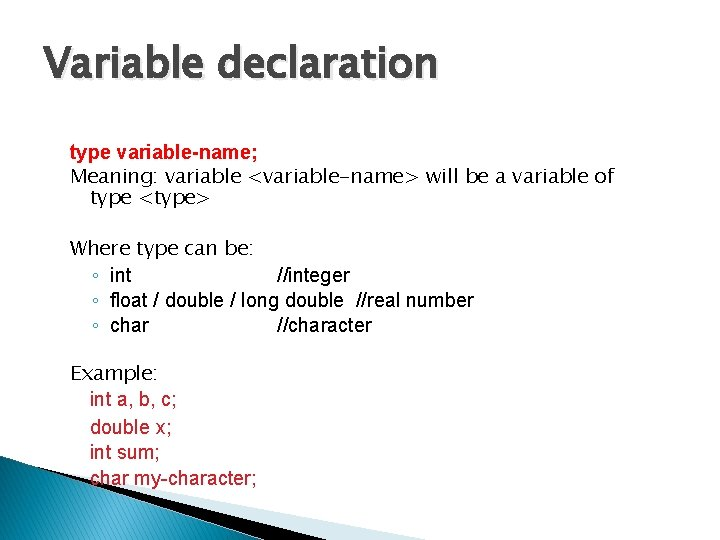Variable declaration type variable-name; Meaning: variable <variable-name> will be a variable of type <type> Where type can be: ◦ int //integer ◦ float / double / long double //real number ◦ char //character Example: int a, b, c; double x; int sum; char my-character;Input statements cin >> variable-name; Extraction operator Meaning: read the value of the variable called <variable-name> from the user Example: cin >> a; cin >> b >> c; cin >> x; cin >> my-character;Output statements cout << variable-name; Insertion operator Meaning: print the value of variable <variable-name> to the user cout << “any message “; Meaning: print the message within quotes to the user cout << endl; Meaning: print a new line Example: cout << a; cout << b << c; cout << “This is my character: “ << my-character << “ he he he” << endl;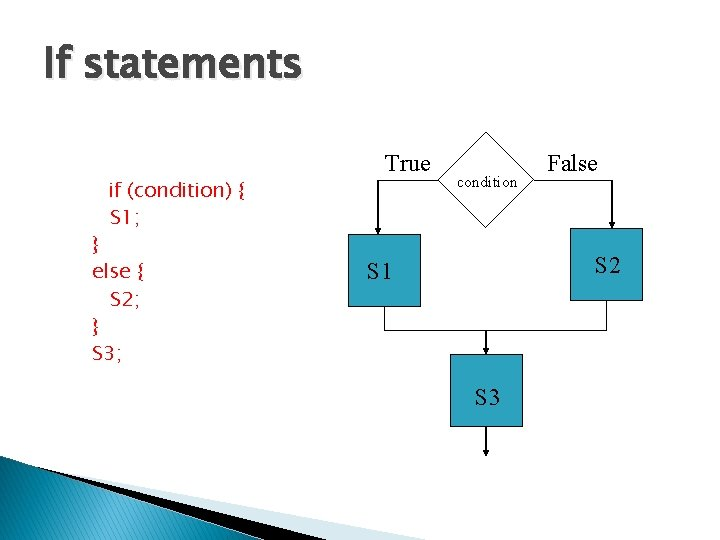If statements if (condition) { S 1; } else { S 2; } S 3; True condition False S 2 S 1 S 3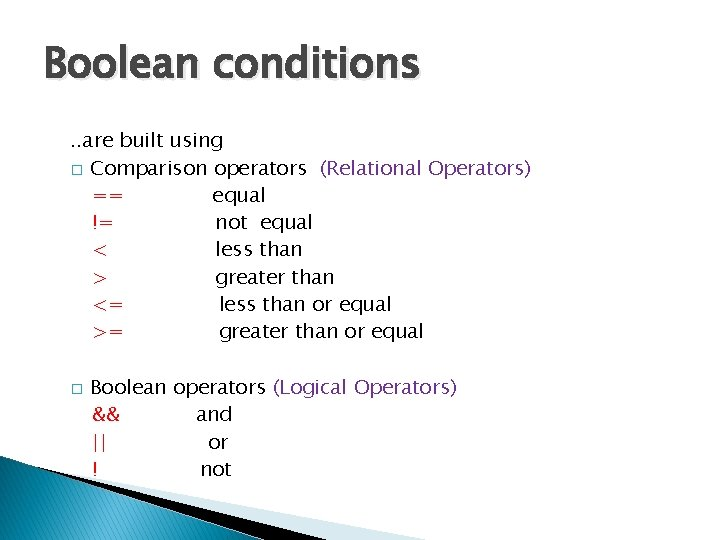Boolean conditions. . are built using � Comparison operators (Relational Operators) == equal != not equal < less than > greater than <= less than or equal >= greater than or equal � Boolean operators (Logical Operators) && and || or ! not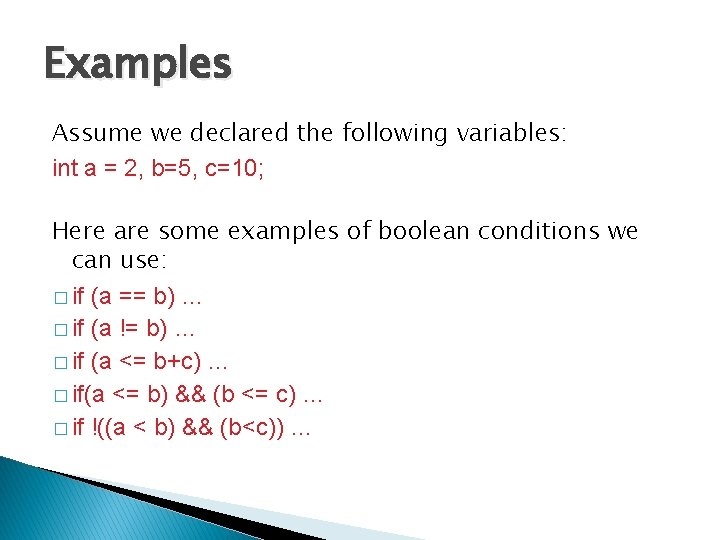Examples Assume we declared the following variables: int a = 2, b=5, c=10; Here are some examples of boolean conditions we can use: � if (a == b) … � if (a != b) … � if (a <= b+c) … � if(a <= b) && (b <= c) … � if !((a < b) && (b<c)) …If example #include <iostream. h> void main() { int a, b, c; cin >> a >> b >> c; if (a <=b) { cout << “min is “ << a << endl; } else { cout << “ min is “ << b << endl; } cout << “happy now? ” << endl; }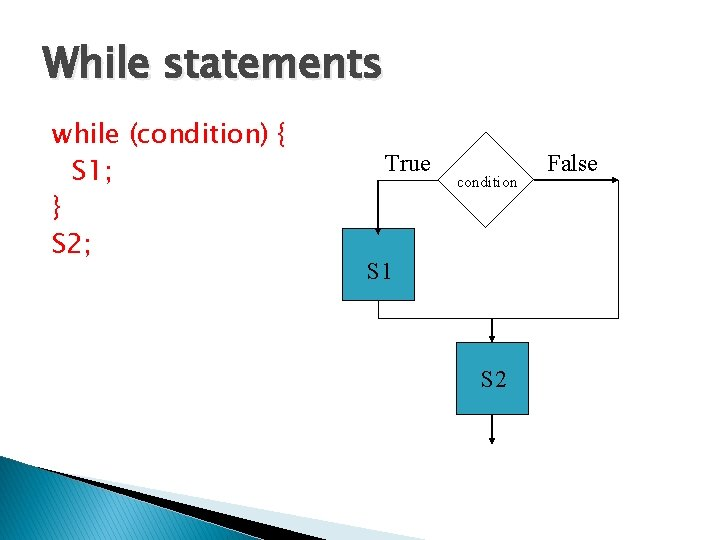While statements while (condition) { S 1; } S 2; True condition S 1 S 2 FalseWhile example //read 100 numbers from the user and output their sum #include <iostream. h> void main() { int i, sum, x; sum=0; i=1; while (i <= 100) { cin >> x; sum = sum + x; i = i+1; } cout << “sum is “ << sum << endl; }Exercise � Write a program that asks the user ◦ Do you want to use this program? (y/n) � If the user says ‘y’ then the program terminates � If the user says ‘n’ then the program asks ◦ Are you really sure you do not want to use this program? (y/n) ◦ If the user says ‘n’ it terminates, otherwise it prints again the message ◦ Are you really sure you do not want to use this program? (y/n) ◦ And so on, every time adding one more “really”.